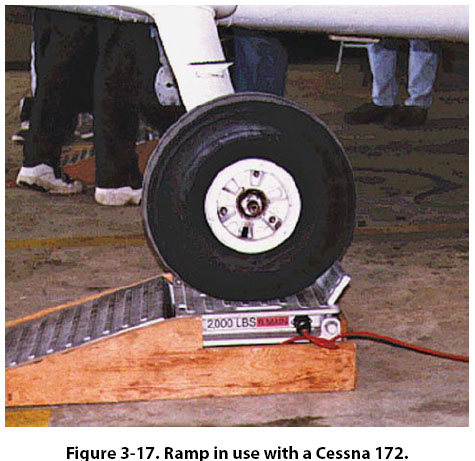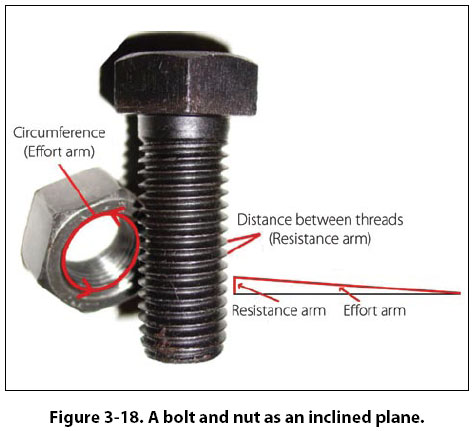Inclined Plane The inclined plane is a simple machine that facilitates the raising or lowering of heavy objects by application of a small force over a relatively long distance. Some familiar examples of the inclined plane are mountain highways and a loading ramp on the back of a moving truck. When weighing a small airplane, like a Cessna 172, an inclined plane (ramp) can be used to get the airplane on the scales by pushing it, rather than jacking it. A ramp can be seen in Figure 3-17, where aCessna 172 right main gear is sitting on an electronic scale. The airplane was pushed up the ramps to get it on the scales. With an inclined plane, the length of the incline is the effort arm and the vertical height of the incline is the resistance arm. If the length of the incline is five times greater than the height, there will be a force advantage, or mechanical advantage, of five. The Cessna 172 in Figure 3-17 weighed 1,600 lb on the day of the weighing. The ramp it is sitting on is 6 inches tall (resistancearm) and the length of the ramp is 24 inches (effort arm). To calculate the force needed to push the airplane up the ramps, use the same formula introduced earlier when levers were discussed, as follows: Effort (E) × Effort Arm (L) = Resistance (R) × Resistance Arm (l) E × 24 in = 1,600 lb × 6 in E = 1,600 lb × 6 in ÷ 24 in E = 400 lb Bolts, screws, and wedges are also examples of devices that operate on the principle of the inclined plane. A bolt, for example, has a spiral thread that runs around its circumference. As the thread winds around the bolt’s circumference, it moves a vertical distance equal to the space between the threads. The circumference of the bolt is the effort arm and the distance between the threads is the resistance arm. [Figure 3-18] Based on this analysis, it can be seen that a fine threaded bolt (more threads per inch) has a greater mechanical advantage than a coarse threaded bolt.A chisel is a good example of a wedge. A chisel might be 8 inches long and only 1/2 inch wide, with a sharp tip and tapered sides. The 8-inch length is the effort arm and the 1/2-inch width is the resistance arm. This chisel would provide a force advantage (mechanical advantage) of 16.
 ©AvStop Online Magazine                                                                                                                                                      Contact Us              Return To Books